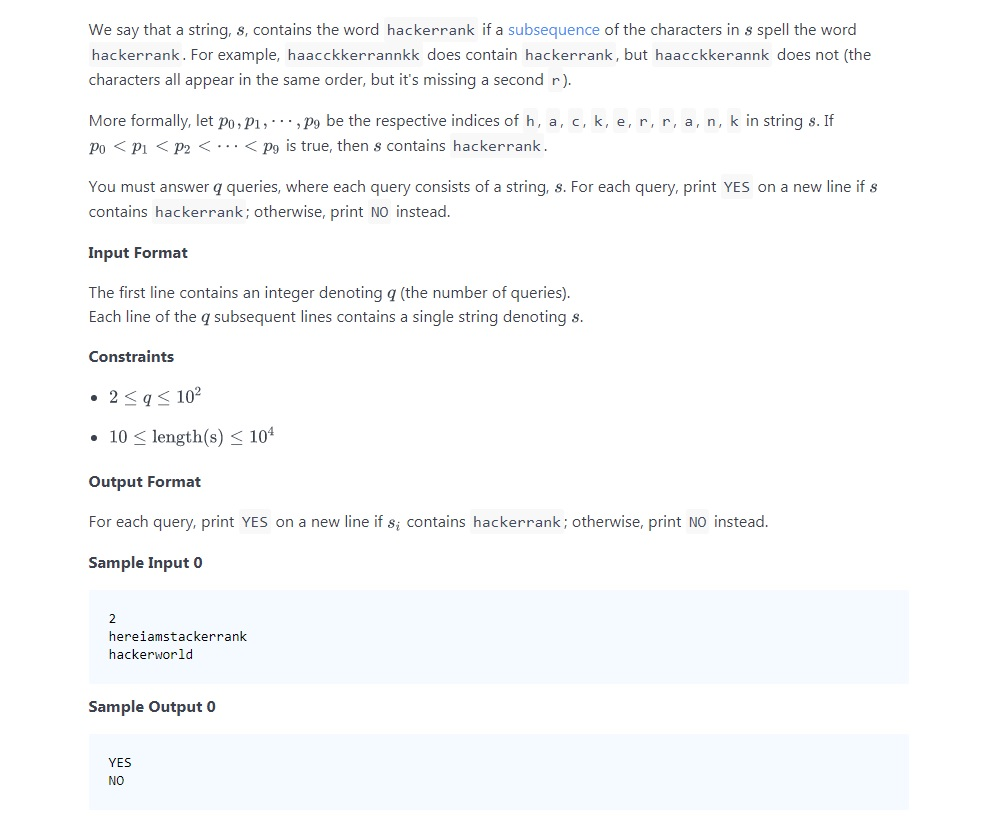# CLEAN CODE: HACKERRANK IN A STRING!

By: Saurav

2018-01-07 22:42:00 UTC

View Problem here: HackerrankThe first thing I thought about was to use the same logic we use in finding the length of common substrings between two string. use. For the given string, go through each character, and view in the input string, while maintaining an array of values where we found the character.

First attempt looks like this:

```def hackerrank_exists(input_str)

if input_str.length < 10
print "NO"
return
end

buffer_array = Array.new(10,100000000000000)

compare_to = "hackerrank"
start = 0

(0..compare_to.length-1).each do |i|
(start..input_str.length-1).each do |j|
if compare_to[i] == input_str[j]
buffer_array[i] = j if buffer_array[i] > j
start = j+1
break
end
end
if buffer_array[i] == 100000000000000
puts "NO"
return
end
end

(0..buffer_array.length-2).each do |k|
if buffer_array[k+1] <= buffer_array[k]
puts "NO"
return
end

end

puts "YES"
return
end ```

But we don't need to keep account of the looping variable in the input string. If we use one loop, and it matches with "hackerrank", it will auto increment.

Attempt 2:

```def hackerrank_exists(input_str)

if input_str.length < 10
print "NO"
return
end

compare_to = "hackerrank"
i = 0

(0..input_str.length-1).each do |j|
if compare_to[i] == input_str[j]
i+=1
end
end

i == 10 ?  "YES" : "NO"

end
```

Time complexity is much reduced and logic is much simpler.
Thanks to the hackerrank forum, I got to learn a pretty sick way of doing it:

Attempt 3:

```def hackerrank_exists(s)
return "NO" if s.length < 10

w = 'hackerrank'.chars
s.chars.each {|c| w.shift if c == w }
puts w.empty? ? 'YES' : 'NO'
end
```

That's the power of ruby! Amazes me each day :)

### Let me know what you think! Twitter: sprakash24octLinkedin

Owned & Maintained by Saurav Prakash

If you like what you see, you can help me cover server costs or buy me a cup of coffee though donation :)